# GMAT Math : Simplifying Algebraic Expressions

## Example Questions

← Previous 1 3 4 5 6

### Example Question #1 : Simplifying Algebraic Expressions

Factor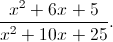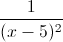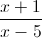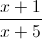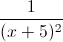Explanation:

Let's first look at the numerator and denominator separately.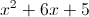: We need two numbers that multiply to 5 and add to 6. The numbers 1 and 5 work. So,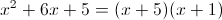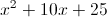: We need two numbers that multiply to 25 and add to 10. The numbers 5 and 5 work. So,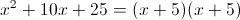Putting this together,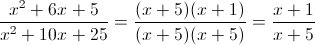### Example Question #1 : Simplifying Algebraic Expressions

Find the solutions to the equation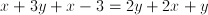.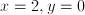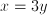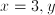is any real number

all real numbers

no solution

no solution

Explanation:

Let's combine like terms.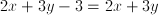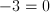, so the equation has no solution.

### Example Question #1 : Simplifying Algebraic Expressions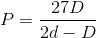Solve for.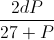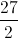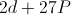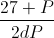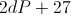Explanation:

You have to isolateby moving around the separate components in the problem.  The steps should go as follows: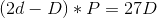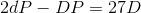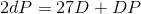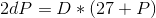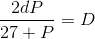### Example Question #1 : Simplifying Algebraic Expressions

Letand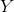be unknown variables. Simplify the following expression: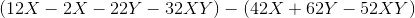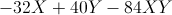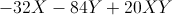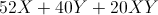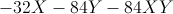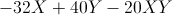Explanation:

To simplify algebraically, we combine like terms. First, we should get the expression in one long string, by removing the parentheses. So remembering the communitive property, the first group in parentheses will have no changes when we remove the parentheses. Sosimplifies to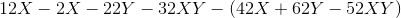However, note the second group in parentheses is being subtracted. So we must invert all the signs in the group to simplify properly. So the previous expression simplifies to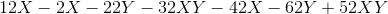Finally we reorder and combine like terms to get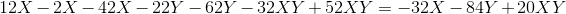### Example Question #1 : Simplifying Algebraic Expressions

A number is divided by 4; its decimal point is then moved to the right 3 places. This is the same as doing what to the number?

Dividing it by 250.

Dividing it by 400.

Dividing it by 4,000.

Multiplying it by 250.

Multiplying it by 2,500.

Multiplying it by 250.

Explanation:

The best way to illustrate the answer to this question is to do these operations to the number 1.

First, divide by 4: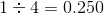Now move the decimal point right three spaces: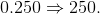This has the effect of multiplying the number by 250.

### Example Question #6 : Simplifying Algebraic Expressions

Which of these expressions is equal to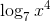?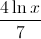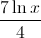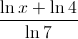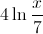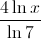Explanation: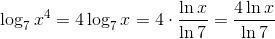### Example Question #1 : Simplifying Algebraic Expressions

The sum of three consecutive integers is 12.  What is the value of the middle integer?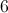Explanation:

Let the value of the first integer be.  This means that the consecutive integers will be,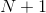, and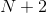.  The sum must be 12 which means that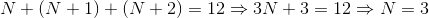Since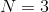the consecutive integers are 3, 4, and 5.  The middle integer is 4.

### Example Question #1 : Simplifying Algebraic Expressions

Solve for.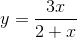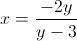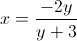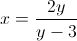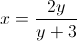Explanation: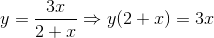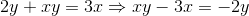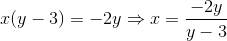### Example Question #1 : Simplifying Algebraic Expressions

Simplify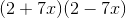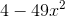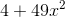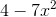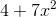Explanation:

Foil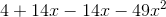### Example Question #1 : Simplifying Algebraic Expressions

Which answer is equivalent to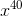?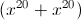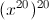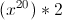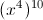Explanation: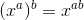Therefore: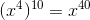← Previous 1 3 4 5 6

### All GMAT Math Resources